Courses

# Test: Centre of Mass- 3

## 30 Questions MCQ Test Physics Class 11 | Test: Centre of Mass- 3

Description
This mock test of Test: Centre of Mass- 3 for Class 11 helps you for every Class 11 entrance exam. This contains 30 Multiple Choice Questions for Class 11 Test: Centre of Mass- 3 (mcq) to study with solutions a complete question bank. The solved questions answers in this Test: Centre of Mass- 3 quiz give you a good mix of easy questions and tough questions. Class 11 students definitely take this Test: Centre of Mass- 3 exercise for a better result in the exam. You can find other Test: Centre of Mass- 3 extra questions, long questions & short questions for Class 11 on EduRev as well by searching above.
QUESTION: 1

### The centre of mass of two particles lies

Solution: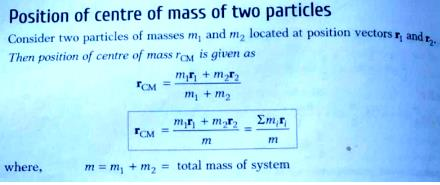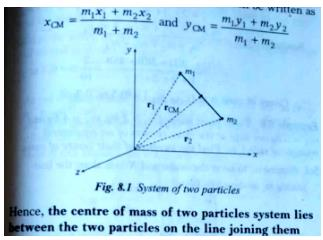QUESTION: 2

### Two billiard balls undergo a head-on collision. Ball 1 is twice as heavy as ball 2. Initially, ball 1 moves with a speed v towards ball 2 which is at rest. Immediately after the collision, ball 1 travels at a speed of v/ 3 in the same direction. What type of collision has occured ?

Solution:

First solve the equation of conservation of momentum which gives us that the relative velocity of approach is equal to the relative velocity of separation. Hence coefficient of restitution is 1. That means the collision is elastic.

mv2 + 2mv3 = 2mv + m0

• v2 = 4v3

• e = 4v3 − v3v – 0

• e = 1

QUESTION: 3

### A ball of 0.1kg strikes a wall at right angle with a speed of 6 m/s and rebounds along its original path at 4 m/s. The change in momentum in Newton- sec is-

Solution:

Mass of the ball = 0.1 Kg

ui  = u = 6 m/s

uf = v = -4 m/s

∴ ΔP = | mΔv |

= | m (v-u) |

= | 0.1 ( -4 - 6 ) |

= | 0.1 ( -10 ) |

= 1

QUESTION: 4

Before a rubber ball bounces off from the floor the ball is in contact with the floor for a fraction of second. Which of the following statements are correct-

Solution:

Obviously the conservation of energy is valid and the direct consequence of it is that KE is converted to compressed PE

QUESTION: 5

A metal ball hits a wall and does not rebound whereas a rubber ball of the same mass on hitting the wall the same velocity rebounds back. It can be concluded that -

Solution:

According to question metal ball doesn't rebound and rubber ball of same mass rebounds, so, rubber ball has change in momentum at least twice as that of metal ball.
mv - (-mv) = 2mv

QUESTION: 6

An object of mass 5 kg and speed 10 ms-1 explodes into two pieces of equal mass. One piece comes to rest. The kinetic energy added to the system during the explosion is-

Solution:

ATQ: Given value mass of object = 5 kg and v = 10 m/s
K.E = 1/2 mv2
1/2 × 5 × 100 = 250 J

QUESTION: 7

A ball is dropped from height 5m. The time after which ball stops rebounding if coefficient of restitution between ball and ground e = 1/2, is

Solution:

The speed of ball just before the first rebound =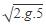Speed of ball just after first rebound = 10 x ½
= 5 m/s
Time taken by the ball before first rebound is 1 sec
Similarly time taken between first and second rebound is 2( 5/10) = ½ sec
Speed of ball after second rebound = 5 x ½
= 2.5 m/s
Hence the time between second and third rebound is ¼ second
Similarly if we find time between more succeeding rebounds we get an infinite GP of common ratio ½ .
Hence the sum is 2 sec.

QUESTION: 8

A ball is dropped from a height h. As is bounces off the floor, its speed is 80 percent of what it was just before it hit the floor. The ball will then rise to a height of most nearly

Solution:

Mgh = 1/2mu2
If v = 0.8u
½ × 0.8u2 × m = mgh'
So,h' = 0.82h
h' = 0.64h

QUESTION: 9

Two billiard balls undergo a head-on collision. Ball 1 is twice as heavy as ball 2. Initially, ball 1 moves with a speed v towards ball 2 which is at rest. Immediately after the collision, ball 1 travels at a speed of v/ 3 in the same direction. What type of collision has occured ?

Solution:
QUESTION: 10

A truck moving on horizontal road east with velocity 20ms-1 collides elastically with a light ball moving with velocity 25 ms-1 along west. The velocity of the ball just after collision

Solution:

Since the system is under no external force momentum is conserved.
Let the mass of truck be M and ball be m, ATQ M>>m
Now, 20M – 25m = mv2 – Mv1 --- (1) where v1 and v2 are the final velocities of the truck and ball respectively
Also, e = ((v2) - (-v1))/((20) - (-25)) = 1
So, v1 + v2 = 45 --- (2)
From equation (1)
20 – 25m/M = m/M v2 – v1
-20 = v1
So, from (2)
Finally, v2 = 65m/s along east

QUESTION: 11

Two balls A and B having masses 1 kg and 2 kg, moving with speeds 21 m/s and 4 m/s respectively in opposite direction, collide head on. After collision A moves with a speed of 1 m/s in the same direction, then the coefficient of restitution is

Solution:

Assuming external forces = 0
1 x 21 – 2 x 4 = 1 x 1 + 2 x v
So, v = 6m/s along the direction of A
Now, e = ((6) - (1))/((21) - (-4))
Hence e = 0.2

QUESTION: 12

When two bodies collide elastically, then

Solution:

In collisions in absence of any external force the momentum is conserved. As the collision is elastic so there will not be any deformation in the shapes of the bodies so there will not be any loss  in energy so the energy will remain constant.

QUESTION: 13

A ball hits the floor and rebounds after an inelastic collision . In this case-

Solution:

When the collision is inelastic the ball doesn't rebond at all but it will stick to the ground. So the momentum of the ball just before and after collision is not conserved and so is it's energy. While considering both the ball and ground as our system the total energy is not conserved since ball losses it's energy but the ground doesn't gain any energy. However momentum will be now conserved because there is no external force acting on our system (gravitational force becomes internal).

QUESTION: 14

In an inelastic collision-

Solution:

In an inelastic collision momentum is conserved but kinetic energy is not conserved, by the virtue of its definition.

QUESTION: 15

A ball strikes a smooth horizontal ground at an angle of 45° with the vertical. What cannot be the possible angle of its velocity with the vertical after the collision. (Assume e <=1).

Solution: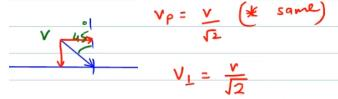The x component will remain same while the y component will become ‘e’ times.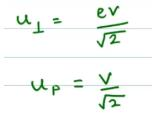Now to find the new angle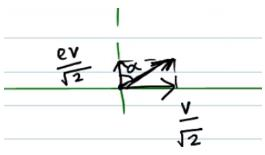tanα = 1/e
Since, e<=1 tanα >= 1 so α >= initial angle

QUESTION: 16

A boy hits a baseball with a bat and imparts an impulse J to the ball. The boy hits the ball again with the same force, except that the ball and the bat are in contact for twice the amount of time as in the first hit. The new impulse equals.

Solution:

J = Ft
1) J = Ft1
2) J = F x 2t1
Hence impulse also gets doubled because impulse is dependent upon time

QUESTION: 17

A system of N particles is free from any external forces

Which of the following is true for the magnitude of the total momentum of the system ?

Solution:
QUESTION: 18

An isolated rail car of mass M is moving along a straight, frictionless track at an initial speed v0 . The car is passing under a bridge when a crate filled with N bowling balls, each of mass m, is dropped from the bridge into the bed of the rail car. The crate splits open and the bowling balls bounce around inside the rail car, but none of them fall out.

Is the momentum of the rail car + bowling balls system conserved in this collision ?

Solution:
QUESTION: 19

Conservation of linear momentum is equivalent to-

Solution:

Conservative of linear moment is when there is no external force on body and this way the case of action and reaction pair cancelling each other by Newton third law of motion.

QUESTION: 20

Two balls are thrown in air. The acceleration of the centre of mass of the two balls while in air (neglect air resistance)

Solution:
QUESTION: 21

A man of mass M stands at one end of a plank of length L which lies at rest on a frictionless surface. The man walks to other end of the plank. If the mass of the plank is, then the distance that the man moves relative to ground is :

Solution:

Let Xm be the displacement of man and Xp of plank
So, Xcom = (MXm + M/3 (-Xp))/(4M/3)
Since, Xcom = 0
So, 3Xm = Xp
Also, Xm + Xp = L

QUESTION: 22

A uniform square plate ABCD has a mass of 10kg. If two point masses of 3 kg each are placed at the corners C and D as shown in the adjoining figure, then the centre of mass shifts to the point which is lie on-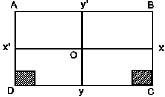Solution:

∵ initial center of mass stays at the center of the square plate. When the mass (20 g) are placed at B and C, the shift along 'y' direction is Zero.
It moves towards the line OY

QUESTION: 23

A particle of mass 3m is projected from the ground at some angle with horizontal. The horizontal range is R. At the highest point of its path it breaks into two pieces m and 2m. The smaller mass comes to rest and larger mass finally falls at a distance x from the point of projection where x is equal to

Solution:

At the time when the particle broke the momentum is conserved as no external force acted
As the vertical component was zero and let say horizontal component was v
Thus we get,
3mv = m.0 + 2m.u
Thus we get u = 3/2 . v
But as there was no change in vertical component of velocity, time of flight does not change. Thus the distance at which the particle would land would be t x v + t x u
Where 2t is time of flight and 2t x v = R
Thus x = R/2 + 3R/4
= 5R/4

QUESTION: 24

Two particles having mass ratio n : 1 are interconnected by a light inextensible string that passes over a smooth pulley. If the system is released, then the acceleration of the centre of mass of the system is :

Solution:

Given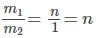Each mass will have the acceleration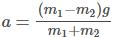However m1 which is heavier will have the will have acceleration a1 vertically down while the lighter mass m2 will have acceleration a2 vertically up → a2=−a1
The acceleration of the center of mass of the system,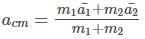Given that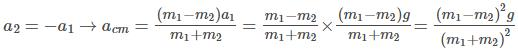Since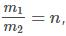diving by m2 and simplifying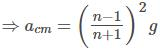Hence c is the correct answer

QUESTION: 25

Which of the following graphs represents the graphical relation between momentum (p) and kinetic energy (K) for a body in motion ?

Solution: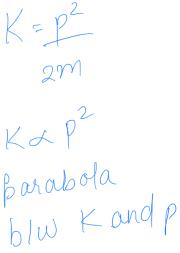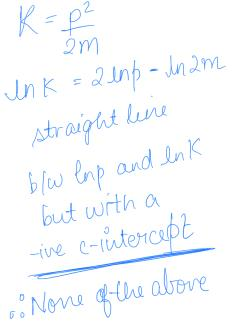QUESTION: 26

A bomb initially at rest explodes by it self into three equal mass fragments. The velocities of two fragments are ( 3+ 2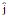) m/s and (__ 4) m/s. The velocity of the third fragment is (in m/s)-

Solution:

Applying law of conservation of momentum,
Mv= m1v1 + m2v2 +m3v3
As initially M is at rest ,v= O
Also m1=m2 =m3 = m (equal masses)
We have m(v1 +v2+ v3) = O
v1+ v2 = -v3
(3i+2j)+(-i-4j) = -v3
3i+2j-i-4j =2i-2j =-v3
v3 = -2i+2j

QUESTION: 27

A stone of mass m1 moving with a uniform speed v suddenly explodes on its own into two fragments. If the fragment of mass m2 is at rest, the speed of the other fragment is-

Solution:

As the stone of mass m1 moving with a constant speed, v,
its momentum is m1v.
When it explodes into 2 fragments, we have m2 the fragment, which remains at rest and hence, its momentum is 0.
The mass of other fragment is m1−m2, if its velocity is V, then its momentum is V(m1−m2).
As momentum is conserved, we should have momentum before the split and after the split as equal and therefore
m1v=0+V(m1−m2)
or, V(m1−m2) =m1v
or, V=m1v/m1−m2

QUESTION: 28

A nucleus of mass number A originally at rest emits a-particle with speed v. The recoil speed of daughter nucleus is :

Solution:

From law of conservation of momentum, we have momentum before collision = momentum after collision
The daughter nucleus has mass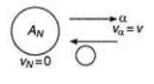The daughter nucleus has mass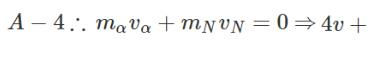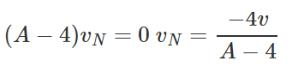The negative sign represents recoil.
Hence A is the correct answer.

QUESTION: 29

A super-ball is to bounce elastically back and forth between two rigid walls at a distance d from each other. Neglecting gravity and assuming the velocity of super-ball to be v0 horizontally, the average force being exerted by the super-ball on each wall is :

Solution:

Change in momentum when ball strikes one wall is:
Δp= −mv0​−m(v0​) = −2mv0
The time it takes to come back and hit the wall again is:
t=−2d/v0
Hence force applied is Δp/t​=mv20/d

QUESTION: 30

A force exerts an impulse I on a particle changing its speed from u to 2u. The applied force and the initial velocity are oppositely directed along the same line. The work done by the force is

Solution:

Impulse, I=change of momentum=2mu−(−mu)=3mu
Work done, W= change of kinetic energy=1/2m(2u)2−1/2mu2
=3/2mu2
=1/2Iu